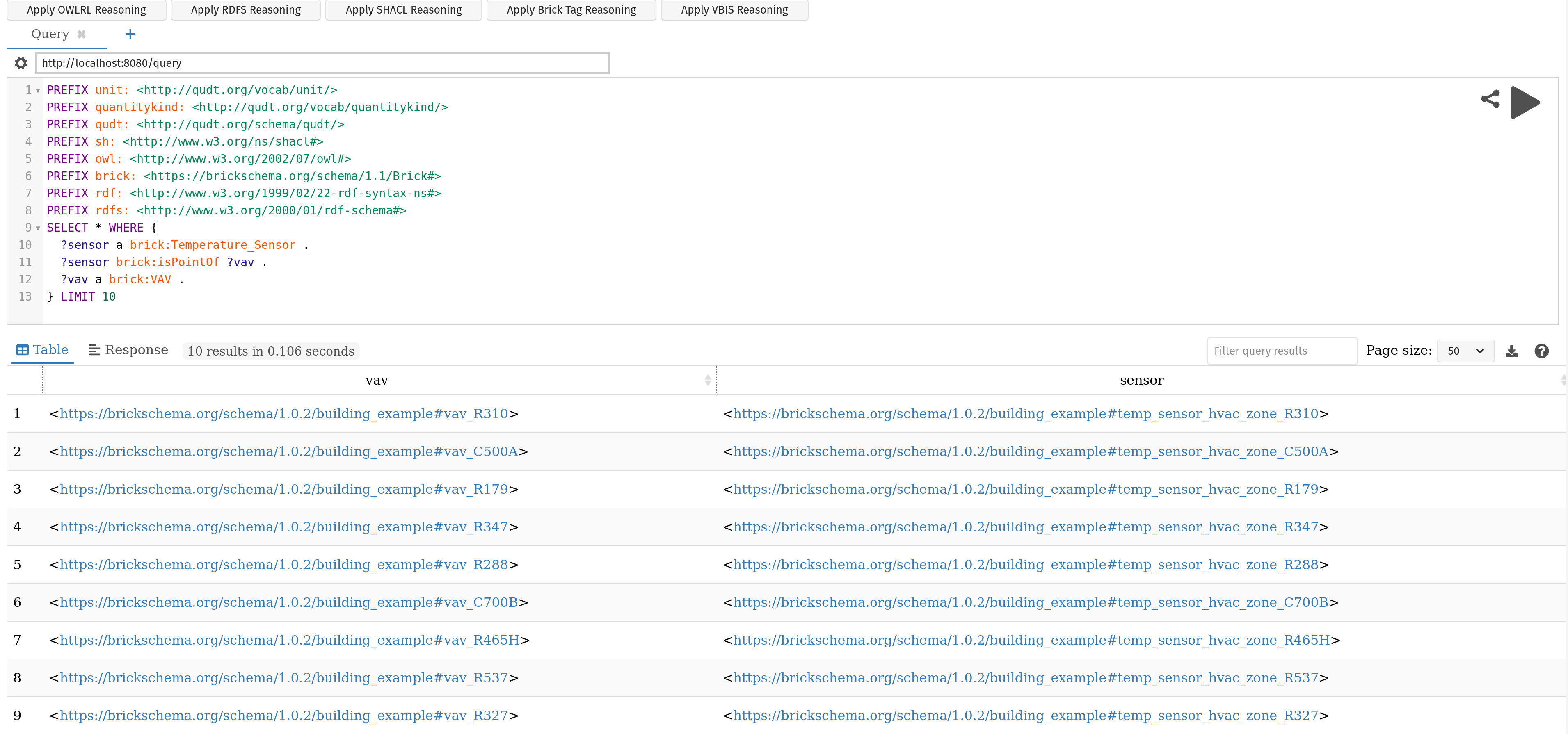# Quick Feature Reference¶

## Web Interface¶

`brickschema` incorporates a simple web server that makes it easy to apply inference and execute queries on Brick models. Call `.serve()` on a Graph object to start the webserver:

```from brickschema import Graph
g = Graph(load_brick=True)
# load example Brick model
g.parse("https://brickschema.org/ttl/soda_brick.ttl")
g.serve("http://localhost:8080") # optional address argument
```## Brick Inference¶

Inference is the process of materializing all of the facts implied about a Brick model given the definitions in the Brick ontology. This process performs, among other things:

• adding in “inverse” edges:
• Example: for all `brick:feeds`, add the corresponding `brick:isFedby`

• annotating instances of classes with their Brick tags:
• Example: for all instances of `brick:Air_Temperature_Sensor`, add the mapped tags: `tag:Air`, `tag:Temperature`, `tag:Sensor` and `tag:Point`

• annotating instances of classes with their measured substances and quantities:
• Example: for all instances of `brick:Air_Temperature_Sensor`, associate the `brick:Air` substance and `brick:Temperature` quantity

• inferring which classes are implied by the available tags:
• Example: all entities with the `tag:Air`, `tag:Temperature`, `tag:Sensor` and `tag:Point` tags will be instantiated as members of the `brick:Air_Temperature_Sensor` class

The set of rules applied to the Brick model are defined formally here.

To apply the default inference process to your Brick model, use the `.expand()` method on the Graph.

```from brickschema import Graph
bldg = Graph(load_brick=True)
bldg.load_file('mybuilding.ttl')
print(f"Before: {len(bldg)} triples")
bldg.expand("owlrl")
print(f"After: {len(bldg)} triples")
```

## Haystack Inference¶

Requires a JSON export of a Haystack model First, export your Haystack model as JSON; we are using the public reference model carytown.json. Then you can use this package as follows:

```import json
from brickschema import Graph
model = json.load(open("haystack-export.json"))
g = Graph(load_brick=True).from_haystack("http://project-haystack.org/carytown#", model)
points = g.query("""SELECT ?point ?type WHERE {
?point rdf:type/rdfs:subClassOf* brick:Point .
?point rdf:type ?type
}""")
print(points)
```

## SQL ORM¶

```from brickschema.graph import Graph
from brickschema.namespaces import BRICK
from brickschema.orm import SQLORM, Location, Equipment, Point
# loads in default Brick ontology
g = Graph(load_brick=True)
# load in our model
g.load_file("test.ttl")
# put the ORM in a SQLite database file called "brick_test.db"
orm = SQLORM(g, connection_string="sqlite:///brick_test.db")
# get the points for each equipment
for equip in orm.session.query(Equipment):
print(f"Equpiment {equip.name} is a {equip.type} with {len(equip.points)} points")
for point in equip.points:
print(f"    Point {point.name} has type {point.type}")
# filter for a given name or type
hvac_zones = orm.session.query(Location)\
.filter(Location.type==BRICK.HVAC_Zone)\
.all()
print(f"Model has {len(hvac_zones)} HVAC Zones")
```